# ARM Mali Profiling Metrics

Some metrics for ARM Mali performance analysis in DS-5 Streamline or MGD.

# DS-5 Streamline

## Mali-470 (Utgard)

GPU Bandwidth = (Words read, master + Words written, master) * Bus Width
Overdraw = Fragments Passed Z/stencil count * Number of Cores / Resolution * FPS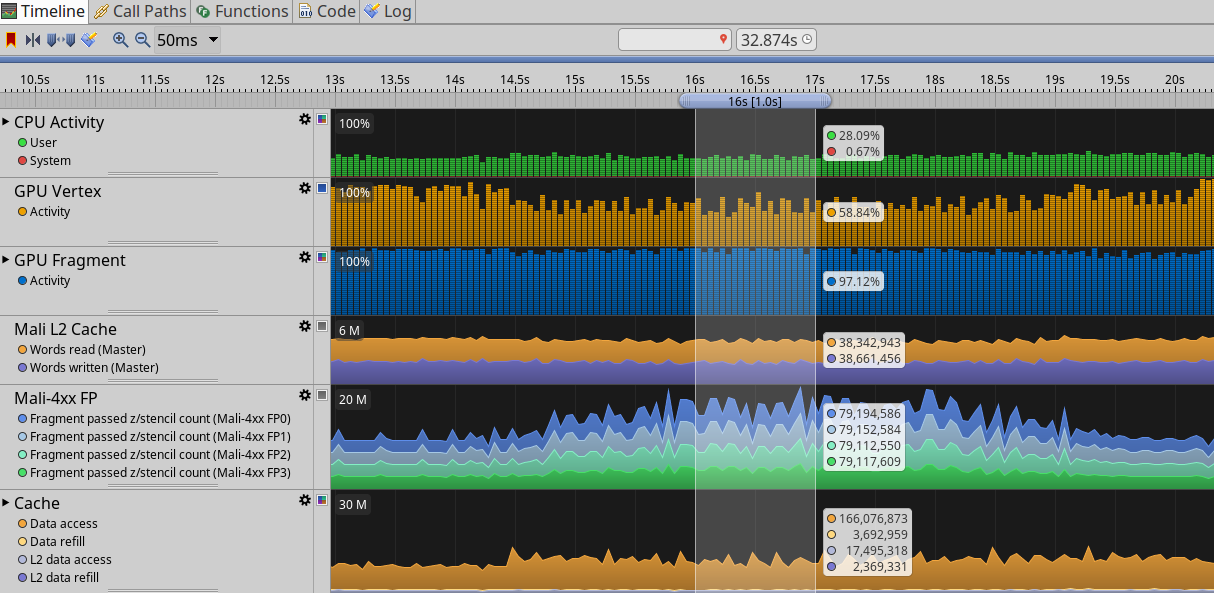GPU Bandwidth = (38342943+38661456) * (128/8) = 1232070384 bytes/s = 1174.99 MB/s Overdraw = (79194586+79152584+79112550+79117609)/(1920x1080) = 152.67

## Mali-T820 (Midgard)

Job Slots

• JS1: vertex, geometry, compute, and tiling.
• JS2: vertex, geometry, and compute.

Fragment Percentage = (JS0 Active / GPU frequency) * 100
Vertex percentage = (JS1 Active / Frequency) * 100
Load Store CPI = Full Pipeline issues / Load Store Instruction Words Completed
GPU Bandwidth = (External read beats + External write beats) * Bus Width
Overdraw = Fragment Threads Started * Number of Cores/ Resolution * FPS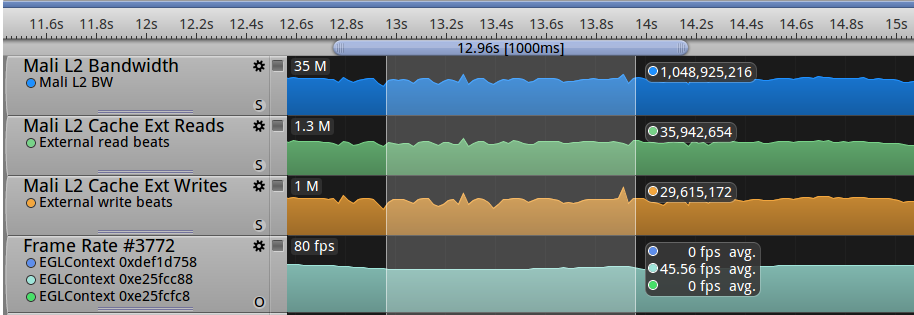GPU Bandwidth = (35942654+29615172) * (128/8) = 1048925216 bytes/s = 1000.33 MB/s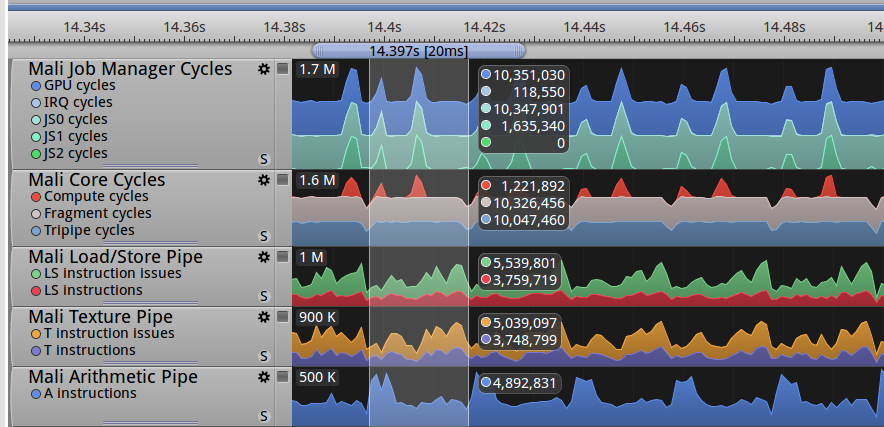Fragment Percentage = (10347901 / 10351030) * 100 = 99.96%
Vertex percentage = ( 1635340 / 10351030) * 100 = 15.79%

# Mali Graphic Debugger(MGD)

GPU Budget
Total vertex cycle count/frame/pixel = vertex cycles / frame buffer pixels
Total fragment cycle count/frame/pixel = fragment cycles / frame buffer pixels
Total cycle count/frame/pixel = fragment cycle + vertex cycle

## Mali-470 (Utgard)

T470MP4@750MHz with 1920x1080 resolution, application rendering framebuffer 512x512.
Total vertex cycle count/frame/pixel = 1224001 / (512 * 512) ~= 4.66 cycles/frame/pixel
Total fragment cycle count/frame/pixel = 6429459 / (512 * 512) = 24.52 cycles/frame/pixel
Total cycle count/frame/pixel = 24.52+4.66 = 29.18 cycles/frame/pixel
vertexCycleBudget = (750M cycles/sec) / (60 frames/sec * 108926) = 114.75 cycles/frame/vertex## Mali-T820 (Midgard)

T820MP3@620MHz with 1920x1080 resolution, application rendering framebuffer 512x512.

Total vertex cycle count/frame/pixel = 919273 / (512 * 512) = 3.5 cycles/frame/pixel
Total fragment cycle count/frame/pixel = 6713456 / (512 * 512) = 25.6 cycles/frame/pixel
Total cycle count/frame/pixel = 29.1 cycles/frame/pixel
vertexCycleBudget = (620M * 3 cycles/sec) / (60 frames/sec * 92141) = 672.88 cycles/frame/vertex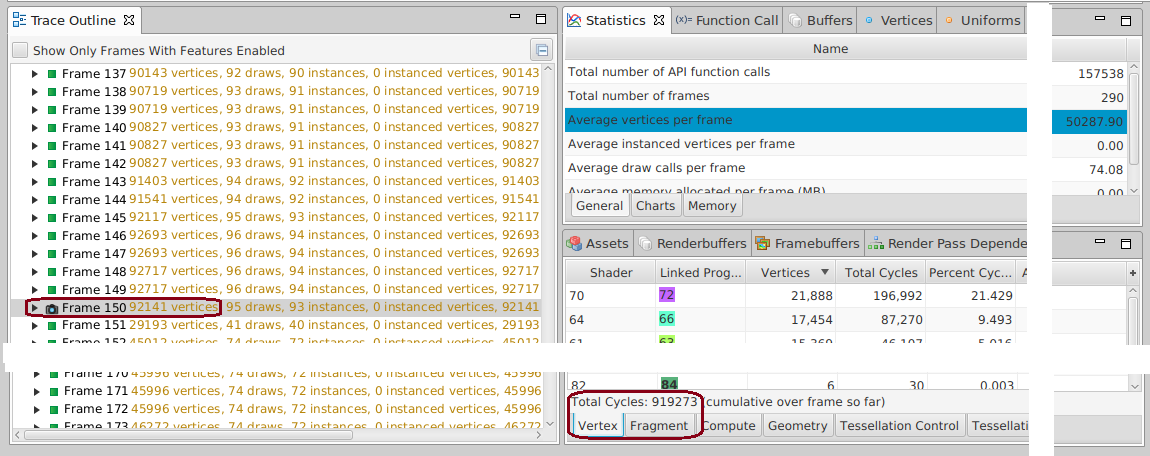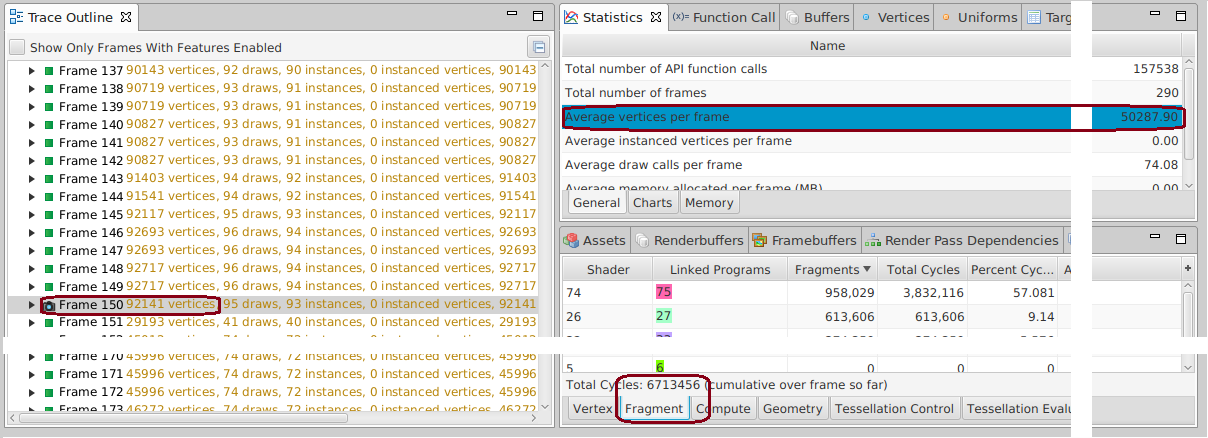References:

Tags:

Updated: